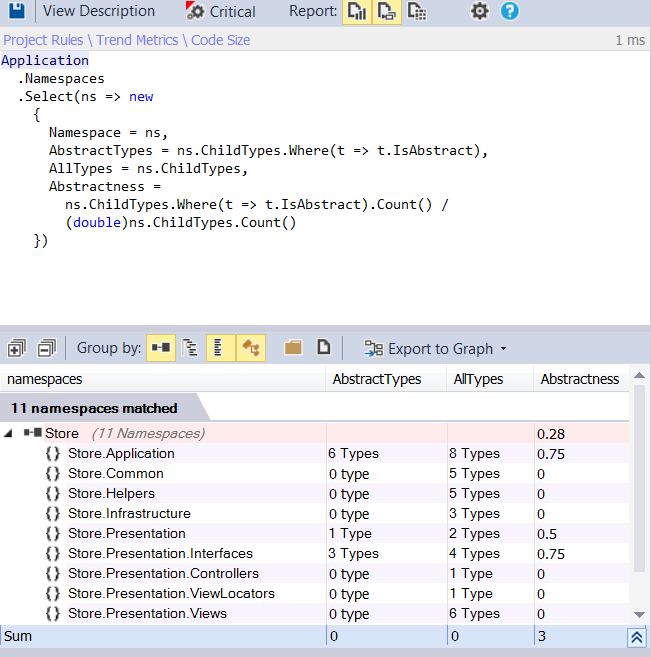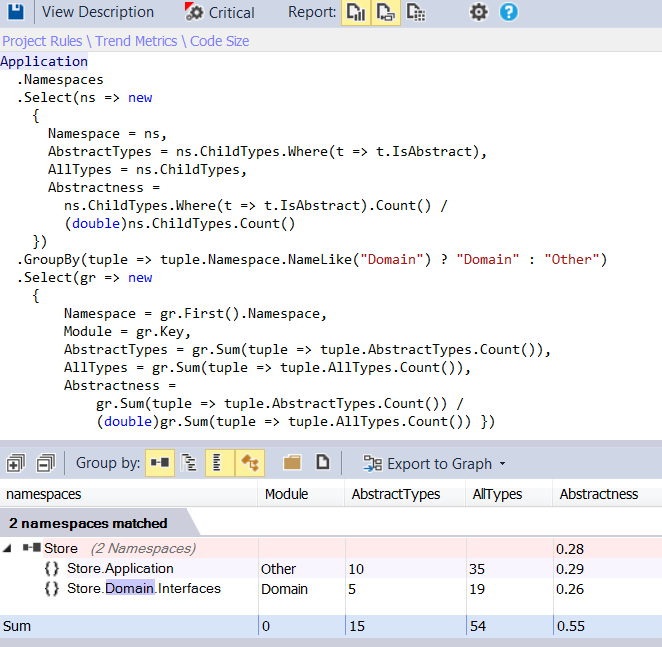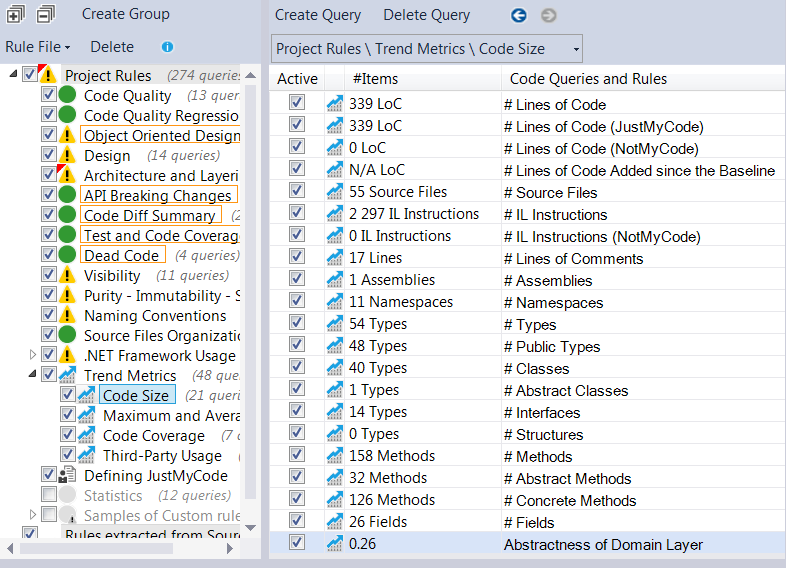# How to Measure Abstractness of Modules Using NDepend and CQLinq

by Zoran Horvat

Our goal here is to define a CQLinq query which tells us the abstractness metric for modules in our application. CQLinq is the specific LINQ query language provided by the NDepend static code analysis tool. We can use CQLinq to analyze, query and measure different aspect of our .NET code.

Abstractness is a metric defined on a set of types. It is the ratio of the number of abstract types (including interfaces) to the total number of types. It tells us how much of the package is abstract. This metric is rarely used alone - it is often coupled with the Instability metric to see how our software package relates to other packages - the more it is abstract, the less instable it should be, or otherwise other packages would get in trouble when this package changes. For more information on software metrics, see Software package metrics at Wikipedia.

In this article we will show how abstractness can be measured using NDepend static code analysis tool using its flavor of LINQ - Code Query LINQ, or short CQLinq. You can learn more on CQLinq directly from the publisher: CQLinq Syntax .

## Defining a Query to Measure Abstractness of Namespaces

Below is the basic CQLinq query which calculates abstractness of each of the namespaces in the application. It is still not the complete query regarding measuring abstractness of modules, but it will form the core of all subsequent queries.

``````Application
.Namespaces
.Select(ns => new
{
Namespace = ns,
AbstractTypes = ns.ChildTypes.Where(t => t.IsAbstract),
AllTypes = ns.ChildTypes,
Abstractness =
ns.ChildTypes.Where(t => t.IsAbstract).Count() /
(double)ns.ChildTypes.Count()
})
``````

When this query is run, it counts types and calculates abstractness of all namespaces in the application. This can be seen from the picture below.Now we have the basic query which separates types by their containing namespaces. Implicitly, it makes an assumption that one namespace is one module - assumption which may be right in very small application, but rarely fits any larger application.

Even in moderately small applications, which consist of only one assembly, some modules contain a namespace together with its child namespaces. It would not be right to take child namespaces as separate modules, as the query above does. However, as you will see, this query can be used as the core of any larger query which is tailored to application at hand.

## One Assembly One Module Assumption

In large applications it is normal to split modules into separate assemblies. In that case, one assembly is one module. We can build on the previous CQLinq query to measure abstractness of all .NET assemblies in the application. Here is the query:

``````Application
.Namespaces
.Select(ns => new
{
Namespace = ns,
AbstractTypes = ns.ChildTypes.Where(t => t.IsAbstract),
AllTypes = ns.ChildTypes,
Abstractness =
ns.ChildTypes.Where(t => t.IsAbstract).Count() /
(double)ns.ChildTypes.Count()
})
.GroupBy(tuple => tuple.Namespace.ParentAssembly)
.Select(gr => new
{
Module = gr.Key,
AbstractTypes = gr.Sum(tuple => tuple.AbstractTypes.Count()),
AllTypes = gr.Sum(tuple => tuple.AllTypes.Count()),
Abstractness =
gr.Sum(tuple => tuple.AbstractTypes.Count()) /
(double)gr.Sum(tuple => tuple.AllTypes.Count()) })
``````

The result of this query is shown in the picture below. The demo application on which we are running this query consists of only one assembly, hence only one row in the result set.## Grouping Namespaces into Modules

In cases where more than one module fits one .NET assembly, we can use the basic CQLinq query above to group namespaces into their corresponding modules. For example, we could have namespaces like Store.Domain.Interfaces and Store.Domain.Implementation. These two namespaces naturally form the Domain Model, which is treated as a single module.

We could build on the previous CQLinq query to calculate abstractness of the Domain Model as a whole:

``````Application
.Namespaces
.Select(ns => new
{
Namespace = ns,
AbstractTypes = ns.ChildTypes.Where(t => t.IsAbstract),
AllTypes = ns.ChildTypes,
Abstractness =
ns.ChildTypes.Where(t => t.IsAbstract).Count() /
(double)ns.ChildTypes.Count()
})
.GroupBy(tuple => tuple.Namespace.NameLike("Domain") ? "Domain" : "Other")
.Select(gr => new
{
Namespace = gr.First().Namespace,
Module = gr.Key,
AbstractTypes = gr.Sum(tuple => tuple.AbstractTypes.Count()),
AllTypes = gr.Sum(tuple => tuple.AllTypes.Count()),
Abstractness =
gr.Sum(tuple => tuple.AbstractTypes.Count()) /
(double)gr.Sum(tuple => tuple.AllTypes.Count()) })
``````

This query appends to the previous query by grouping the types by their containing namespaces. All types belonging to namespaces containing Domain in their name are taken as part of the Domain Model. Other types are separated from them.

After that first, grouping step, we are calculating the abstractness metric once again, this time counting all types together before calculating the ratio.

When this query is run, Domain types are grouped together and all others are grouped separately. The result can be seen in the picture below.## Defining a Trend Metric to Track Abstractness

Now that we have all these queries at hand, we are free to define a trend metric. NDepend supports custom trend metrics as a specific kind of a query - a query returning a number. NDepend then uses this query to build a series of numeric values which it can draw as a chart. Further on, developers can use such charts to view progress made over a longer period of time spent on the project.

Regarding the abstractness metric, we can reuse the queries we have built along the route to turn them into a query returning a single number. For example, below is the trend metric which calculates abstractness of types defined in namespaces containing Domain in their name.

``````// <TrendMetric Name="Abstractness of Domain Layer" Unit="" />
Application
.Namespaces
.Select(ns => new
{
Namespace = ns,
AbstractTypes = ns.ChildTypes.Where(t => t.IsAbstract),
AllTypes = ns.ChildTypes,
Abstractness =
ns.ChildTypes.Where(t => t.IsAbstract).Count() /
(double)ns.ChildTypes.Count()
})
.GroupBy(tuple => tuple.Namespace.NameLike("Domain") ? "Domain" : "Other")
.Select(gr => new
{
Namespace = gr.First().Namespace,
ModuleName = gr.Key,
AbstractTypes = gr.Sum(tuple => tuple.AbstractTypes.Count()),
AllTypes = gr.Sum(tuple => tuple.AllTypes.Count()),
Abstractness =
gr.Sum(tuple => tuple.AbstractTypes.Count()) /
(double)gr.Sum(tuple => tuple.AllTypes.Count()) })
.Where(tuple => tuple.ModuleName == "Domain")
.Select(tuple => tuple.Abstractness)
.Max()
``````

This query builds on one of the previous queries and adds final three statements to make it the trend metric. These three statements actually isolate the Domain module and then pick its Abstractness metric calculated previously. The last operation, taking the maximum of values, is there due to certain limitations posed by NDepend. We would normally use the Single operation after Select, but NDepend doesn’t support that so we take the Max operation as the second best.

When faced with narrowly scoped goal, we can surely simplify the LINQ query. The query above is too general for the narrow purpose of measuring Domain Layer abstractness and we can greatly simplify it for the purpose. Here it is:

``````// <TrendMetric Name="Abstractness of Domain Layer" Unit="" />
Application.Namespaces
.WithNameLike("Domain")
.ChildTypes()
.Where(t => t.IsAbstract)
.Sum(t =>
1 / (double)Namespaces.WithNameLike("Domain").ChildTypes().Count())
``````

This query returns the same value as the previous one.

Once the trend metric is defined (note the first line of it, giving the name and the unit), NDepend will offer it in the list of code metrics as any other built-in metric. Picture below shows the list of metrics. If you take a look at the very bottom of the list, you will see the Abstractness of Domain Layer metric which was just defined using the query above. This view also gives us the latest value obtained from the metric query - 0.26 in this particular case.## Conclusion

In this article we have tackled the question of defining one particular code metric - Abstractness metric - for the modules in our project. It is a good idea to use such metrics as the long running project unfolds.

It is also a good idea to define trend metrics and to let NDepend draw trend charts that can be included in analysis reports. Viewing the gradual changes to these metrics from time to time could give valuable early signs that design is sliding off the tracks or be used as insurance that the design is continuing as planned.